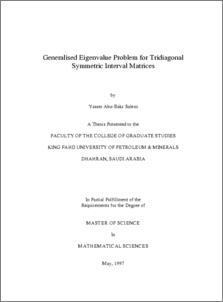# Generalised eigenvalue problem for tridiagonal symmetric interval matrices.

Salem, Yasser Abu-Bakr (1997) Generalised eigenvalue problem for tridiagonal symmetric interval matrices. Masters thesis, King Fahd University of Petroleum and Minerals.Preview
PDF
1775Kb

-

## English Abstract

Analgorithm to determine the eigenintervals of the generalized eigenvalue problem A¹ x =λB¹ x, where (A¹, B¹) is a pair of real tridiagonal symmetric interval matrices is presented. The eigenintervals are exactly calculated. The algorithm requires twice as much computational effort as the Sturm algorithm which is used for real point matrices.

Item Type: Thesis (Masters) May 1997 Completion Math College Of Sciences > Mathematical Science Dept Salem, Yasser Abu-Bakr El-Gebeily, Mohamed Selim, Shokri Z. and El-Gindi, Mohamed 10306 KFUPM ePrints Admin 22 Jun 2008 17:01 26 Apr 2011 08:09

Repository Staff Only: item control page